# Learning Goal: To review the meaning ofcapacitance and ways of changing the capacitance of aparallel-plate capacitor

Learning Goal: To review the meaning ofcapacitance and ways of changing the capacitance of aparallel-plate capacitor.

Capacitance is one of the central concepts in electrostatics.Understanding its meaning and the difference between its definitionand the ways of calculating capacitance can be challenging atfirst. This tutorial is meant to help you become more comfortablewith capacitance. Recall the fundamental formula for capacitance: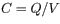where C is the capacitance in farads,Q is the charge stored on the plates incoulombs, and V is the potential difference (or voltage)between the plates. In the following problems it may help to keepin mind that the voltage is related to the strength of the electricfield E and the distance between theplates, d, by
V = Ed.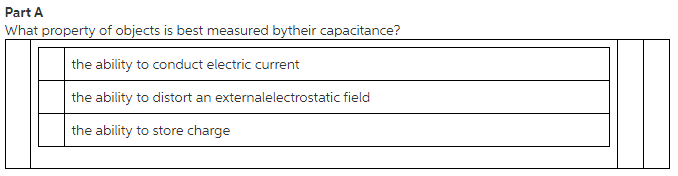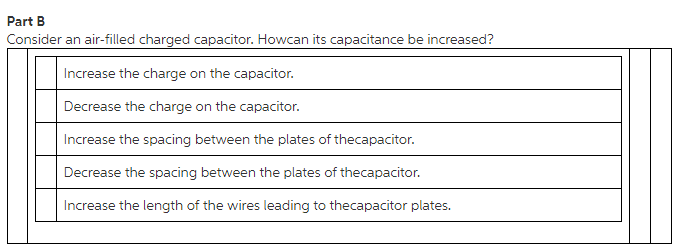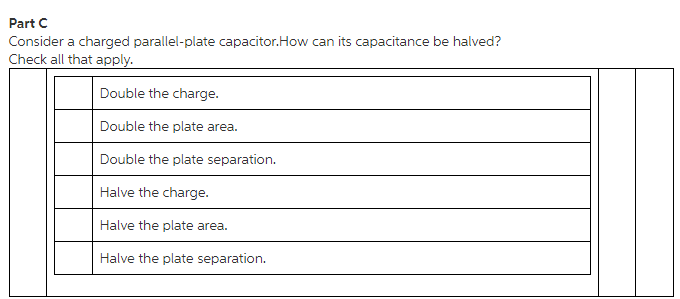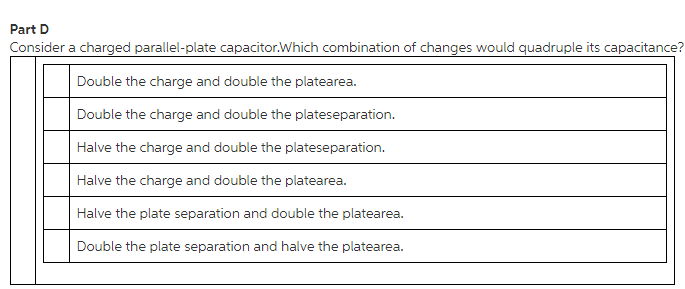Concepts and reason
The concept related to solve this problem is the capacitance of the capacitor.
First explain which property of the objects is best measured by their capacitance. Next, explain the how the capacitance can be increased in an air filled charged capacitor. After that Explain how the capacitance is halved. Finally, explain which combination of changes would quadruple its capacitance.

Fundamentals
Capacitance is the ability of a body to store an electric charge. The ratio of the charge to the potential difference is called capacitance.
The capacitance of the capacitor is,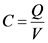Here, C is the capacitance, Q is the charge stored in the capacitor, and V is the potential difference between the plates.

Part A
The property that best measured by the capacitance is the amount of charge stored on the capacitor. When the potential difference applied between the ends of the conductor, then the amount of charge stored in the capacitor is the measure of capacitance. It is given as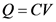Part A
The property that best measured by the capacitance is the amount of charge stored on the capacitor.
Capacitor has the ability to store energy on the form of electric charge that producing a potential difference across the plates of the capacitor.

Part B
The capacitance of the capacitor is,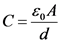Here, A is the area of the plates,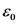is the permittivity of free space, and d is the distance between the plates.
From this formula, the capacitance is increased by increasing the area of the plate and decreasing the distance between the plates.

Part B
The capacitance of the capacitor increased by the decreasing the spacing between the plates of the capacitor.
The capacitance of the capacitor is directly proportional to the area of the plates and inversely proportional to the distance between the plates.

Part C
By reducing the area of the plate to half, the capacitance of the capacitor can be made half. By doubling the distance between the plates, then the capacitance of the capacitor is halved.

Part C
The capacitance of the capacitance can be halved if the area of the plates is half. The capacitance of the capacitance can also be halved if the separation between the plates is double.

Explanation: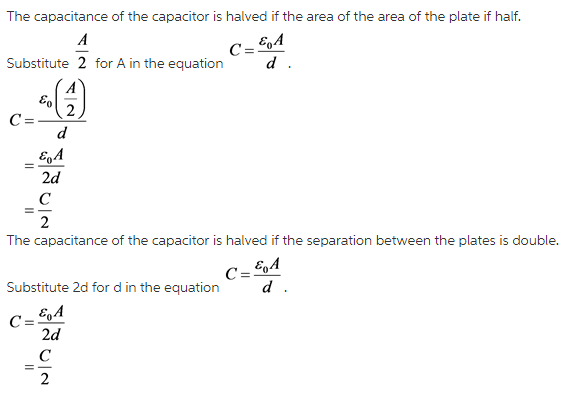Part D
The capacitance of the capacitor can be quadrupled by doubling the plate area and reducing the separation between the plates to half in a charged parallel plate capacitor.

Part D
The capacitance of the capacitor can be quadrupled if the plate separation is half and double the area of the plate.

Explanation:The capacitance of the capacitor is quadrupled if the area of the plates is double and the separation between the plates is halved.

Part A
The property that best measured by the capacitance is the amount of charge stored on the capacitor.
Part B
The capacitance of the capacitor increased by the decreasing the spacing between the plates of the capacitor.
Part C
The capacitance of the capacitance can be halved if the area of the plates is half. The capacitance of the capacitance can also be halved if the separation between the plates is double.
Part D
The capacitance of the capacitor can be quadrupled if the plate separation is half and double the area of the plate.